# LOOKUP function Blank resultHello everyone,

I am currently working on a model where i am using some data i imported in formulas to create some relevant data for a YTD model. I am trying to implement the following excel formula :  IFERROR(VLOOKUP(A2;'TCD CRISTALLINE'!H:I;2;0);""). So in the first part of the implementation i started off by trying to use uniquely the LOOKUP formula, and so :

• I created a list and imported all the data that i'll be needing into it (Composant list screenshot.png)
• I created the module and formatted the data types with the dimensions ill be using (ACHATS YTD ACCESS&PRECIOUS module screenshot.png)

The column where i need to insert the formula above is the "ref pierre de centre" column, and so i used the following formula : COMPOSANT.Composant[LOOKUP: Modèle], which should simply look for the composant from the list above corresponding to each "modèle" item i have. However, i am not getting any values, and the whole column is blank. I also tried to create a module , take the list i needed as a dimension in a new module (Composant module screenshot.png) and import to it the "composants" data, and then use the formula Composant module.Composant[LOOKUP: Modèle] (module test 2 screenshot.png), but i get always the same result.

Yassine

Tagged:

•Hi,

You are getting blank, because the lookup line item you used doesn't have any data.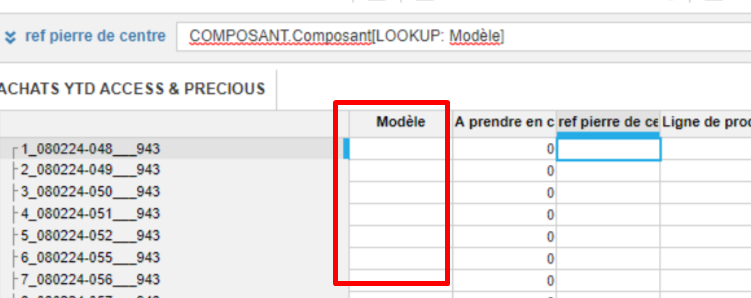Populate the Modele line item, then you will get the data.

Thanks,

Vignesh M

•I did populate the module with data, and yet its the same problem, even in the other module.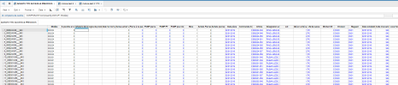•Hi Yassine,

Search "080224" in your composant list, I think Composant Property is blank for this record. Please check.

~Vignesh M

•Can you please confirm that the line item 'Modele' is Composant List formatted. It has to be else the formula wouldn't work properly. You need a connection/relation between your module (Source) and the list (Target).

Regards,

Kavin.

•Not all cells contain the data, indeed the "080224" doesn't have a corresponding value of "ref pierre de centre", but for example the "J1MK00" does in my excel model. however, its blank in my Anaplan model.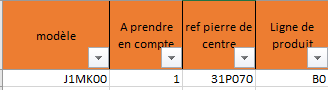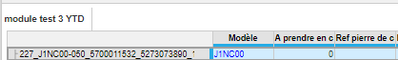•the "Modele" is text formatted since i am getting the data in it using the formula : IF LEFT(Article, 1) = "W" THEN Article ELSE LEFT(Article, 6) in wich i am extracting the first 6 characters from my "Article" column, filled with data i imported.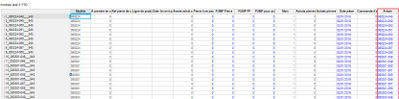•Can you please convert the 'modele' line item to List formatted one(Composant). Create another line item with 'Composant list' formatted and write the formula 'FINDITEM('COMPOSANT', Modele)' then do a lookup based on this newly created one.

I hope this solves your concern.

Let me know if you have any questions.

Regards,

Kavin.

•I get the following error when trying to convert the Module line item to a Composant list format :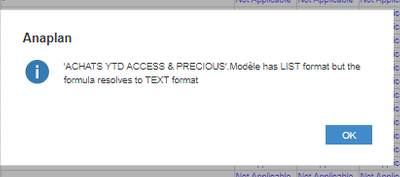and its because i am using this formula to fill data in the modele line item : IF LEFT(Article, 1) = "W" THEN Article ELSE LEFT(Article, 6). What should i do in this case ?

•Try this, FINDITEM('Composant',IF LEFT(Article, 1) = "W" THEN Article ELSE LEFT(Article, 6)).

Kavin.

•I get blank results? the "module 1" is like you instructed COMPOSANT list formatted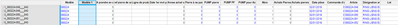Thanks again for the help

•Hi Yassine,

Try this,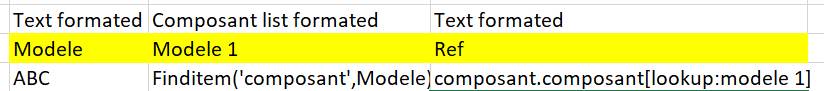Also check, whether "080224" is present in your list or not.

~Vignesh M